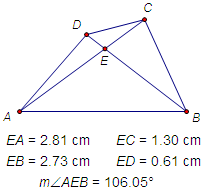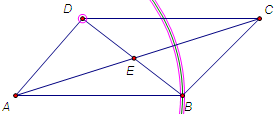﻿ Menus > Edit Menu > Split/Merge > How to Use Split and Merge to Explore Constructions

# How to Use Split and Merge to Explore Constructions1.To begin, construct quadrilateral ABCD, its two diagonals, and the point of intersection E of the diagonals. Then measure the distances and angle shown in the figure at right. Drag the vertices around to see if there’s any relationship between the various measurements.2.Now turn the quadrilateral into a parallelogram. Construct two parallel lines as shown at right: one through D parallel to the segment connecting A and B, and another through B parallel to the segment connecting A and D. Also construct the intersection of the parallels.3.Select both point C and the intersection, and choose Edit | Merge Points to merge the vertex with the intersection point.4.Hide the parallel lines. Then drag vertices A, B, and D. How are the measurements now related?5.Next turn the quadrilateral into a rectangle by makingBAD into a right angle. Construct a perpendicular as shown at right and merge point D to the perpendicular. Again, drag the vertices and watch the measurements. Do you notice anything different?6.Finally, change your quadrilateral into a rhombus. First split D from the perpendicular. Then construct a circle centered at A and passing through B, and merge D to the circle. Drag the vertices once more to see what relationships the measurements now reveal.